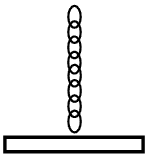# What a chain! 2A very flexible uniform chain of mass $M$ and length $L$ is suspended vertically such that its lower end just touches the surface of the table.

When the upper end of the chain is released, it falls with each link coming to rest the instant it strikes the table.

Find the force exerted by the chain on the table at the moment when $y$ part of the chain has already come to rest on the table.

Your answer is of the form $\dfrac { aMgy }{ L }$, where $a$ is a positive integer. Submit the value of $a$ as your answer.

Details and Assumptions**:

• The fallen part don't interfere with the falling one.

• The figure is not to scale.

×